# Modified Secant Method for Reduction in Number of Iterations

Modified Secant Method for Reduction in Number of Iterations
Department of Mechanical Engineering, National Institute of Technology, Raipur Chhattisgarh 492010, India

Corresponding Author Email:
akshukla.me@nitrr.ac.in
Page:
376-382
|
DOI:
https://doi.org/10.18280/mmep.100144
26 July 2022
|
Accepted:
25 December 2022
|
Published:
28 February 2023
| Citation

OPEN ACCESS

Abstract:

The present study considers an updated solution for an improved secant method. The study presents certain changes to improve the existing method with given conditions for the roots of the non-linear equation where f(x)=0 and the function is continuous. Here an improved method has been developed that starts with the basic secant method, and after considering these conditions, an efficient solution is obtained. This modified method will increase the convergence rate faster than the standard secant method by performing a few iterations. The present solution confirmed that the proposed method is effective compared to the standard secant method. The present work developed based on additional conditions derived from the standard secant method and is responsible for reducing the number of iterations.

Keywords:

non-linear equation, secant method, modified secant method, numerical solution

1. Introduction

The present work aims to find improvement in the non-linear equation solutions that require many iterations. The secant method is one of the best, considering its faster convergence rate than the Regula Falsi and Bisection method. The formulation uses three successive points of the iteration instead of just two, and the order of convergence is 1.839 . An improved Newton Raphson’s method has been developed, where a definite area integral is approximated as trapezoidal area in place of the rectangular area . A zero-finding method is used to solve non-linear equations, which is most efficiently used with the traditional iterative method in which the order of convergence is improved . The leap-frog Newton's method has been developed using Newton's method as an intermediate step. At a simple root, the order of convergence is cubic, and the computational efficiency is lower but still quite comparable to Newton's approach . To approximate a locally unique solution of a non-linear equation in Banach spaces, the idea uses Lipschitz and center–Lipschitz instead of just Lipschitz conditions in the convergence analysis . An improved Regula-Falsi method with an order of convergence of 3 combines the usual Regula-Falsi method and a Newton-like method to solve for f(x) = 0 . A novel approach for solving non-linear equations that is similar to the Secant method. The convergence analysis reveals that the asymptotic order of convergence of this method is $(1+\sqrt{3})$ . A modified secant method is analysed under the hypothesis of second-order derivatives (Lipschitz continuous) with an error analysis . An improved Regula Falsi (IRF) method based on the classic Regula-Falsi (RF) method has been tested considering many numerical examples and the results confirm that the proposed method performs well compared to the traditional Regula-Falsi method . Another novel class of spectral conjugate gradient algorithms studied to achieve high-order accuracy in predicting the second-order curvature . To obtain similar convergence to Newton's approach without analyzing any derivatives, a generalization of the Secant method to semi-smooth equation is suggested . The Newton-Raphson method makes it clear that the correction needed to obtain the right value of the root decreases as the derivative f(x) increases . To increase the Secant method's applicability, changes have been made to the resolution of a linear system in each step required to use the secant approach for multiple matrix multiplications . To solve a non-linear equation, modification has been done using the secant method, which involves the development of the inverse of the first-order divided differences of a function of several variables at two points . Although the Newton-Raphson method converges quickly close to the root, its global characteristics are poor . Several researchers tried to reduce the number of iterations, which reduces the computational cost of the Secant method. The present paper aims to find an improved method that is faster than the standard Secant method. According to the present study, some changes to the Secant method's standard conditions are required. These conditions are as follows:

Condition 1: - Either $f\left(x_a\right) * f\left(x_b\right)$  or $f\left(x_a\right) * f\left(x_b\right)>0$

where, xa and xb are two initial guesses, and f(xa) and f(xb) are function value. It demonstrates that the signs of f(xa) and f(xb) can be opposite or identical. The Modified Secant method also satisfied the requirement mentioned above, which is true.

Condition 2: - After making two initial estimations, xa and xb, the first root value can be calculated using the standard Secant formula. If the value obtained is x1, then the equation has a root only if f(x1)=0; otherwise, f(x1)>0 or f(x1)<0. Now, the formula for the following iterations includes two values, one of which is the new iteration value, i.e., x1, and the other one is immediately before it, i.e., xb but not xa.

This Modified Secant approach no longer allows condition- 2. The entire fundamental is based on condition 2. The Modified Secant approach has been developed in this paper, which will also show the transformation of the standard Secant method into the Modified Secant method. The reader must have an understanding of triangular properties. Consider Figure 1, where xa and xb are the two roots. Draw a chord that joins two points, f(xa) and f(xb), due to this chord, we now have two triangles i.e., $\Delta\left(f\left(x_a\right), x_1, x_a\right)$  and $\Delta\left(f\left(x_b\right), x_1, x_b\right)$ , we can use similar triangular properties to solve the standard Secant formula. As we know, triangular properties are:

• The two triangles must be of the same shape, and their size may vary,
• Each pair of corresponding angles are equal,
• Corresponding sides are in the same proportion.

## 1.pngFigure 1. Function curve with two initial guesses i.e., xa and xb

Figure 1 shows the two function values, f(xa) and f(xb)are of opposing signs.

The two most recent root approximations are used to find the next approximation in the standard Secant technique .

2. Description of the Problem

As the standard Secant method uses succession roots to check the function value. The improved Secant technique used condition 2 and considers, instead of focusing on two recent values, i.e., xa and xb to calculate a new approximate value, i.e., x1. Suppose to emphasis the function value by repeatedly checking the function value after each new iteration by using Modified Secant method conditions (A and B). In that case, the number of iterations reduces significantly. The standard Secant method for the 2nd iteration after the first iteration formula is given below.

$x_2=\frac{\left(x_b * f\left(x_1\right)-x_1 * f\left(x_b\right)\right)}{f\left(x_1\right)-f\left(x_b\right)}$    (1)

The Modified Secant approach for the 2nd iteration of the formula, Conditions (A and B), is as follows:

If $a b s\left(f\left(x_a\right)\right)-a b s\left(f\left(x_1\right)\right)<a b s\left(f\left(x_b\right)\right)-a b s\left(f\left(x_1\right)\right)$ use xa with x1 for 2nd iteration(A).

If $a b s\left(f\left(x_b\right)\right)-a b s\left(f\left(x_1\right)\right)<a b s\left(f\left(x_a\right)\right)-a b s\left(f\left(x_1\right)\right)$ use xb with x1 for 2nd iteration(B).

3. Methodology

The Modified Secant method applied immediately after the first iteration, and the starting formulation remains the same for Regula Falsi, the Secant method, and the Modified Secant method. Let two initial guesses be, $x_a$ and $x_b$(the function values of both negative, and positive, or one negative and one positive, have no effect on the initial formula) . By employing a similar triangular property. The first iteration formula is as follows: Refer to Figure 1.

## 2.png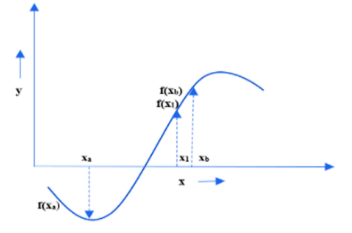Figure 2. Function curve with three roots, i.e., xa, xb and x1 for finding x2

$\begin{gathered}\frac{\left(x_1-x_a\right)}{\left(-f\left(x_a\right)\right)}=\frac{\left(x_b-x_1\right)}{f\left(x_b\right)} \\ f\left(x_b\right) * x_1-f\left(x_b\right) * x_a=\left(-f\left(x_a\right) * x_b\right)+f\left(x_a\right) * x_1 \\ f\left(x_b\right) * x_1-f\left(x_a\right) * x_1=\left(-f\left(x_a\right) * x_b+f\left(x_b\right) * x_a\right. \\ \left(f\left(x_b\right)-f\left(x_a\right)\right) * x_1=f\left(x_b\right) * x_a-f\left(x_a\right) * x_b \\ x_1=\frac{\left(x_a * f\left(x_b\right)-x_b * f\left(x_a\right)\right)}{\left(f\left(x_b\right)-f\left(x_a\right)\right)}\end{gathered}$    (2)

For the second iteration, use the conditions (A and B): Since the modified approach will compute after the first iteration, it's critical to illustrate the second iteration formula. Refer to Figure 2.

If $a b s\left(f\left(x_a\right)\right)-a b s\left(f\left(x_1\right)\right)<a b s\left(f\left(x_b\right)\right)-a b s\left(f\left(x_1\right)\right)$ use xa with x1 for new iteration (A).

$\begin{gathered}\frac{\left(x_2-x_a\right)}{\left(-f\left(x_a\right)\right)}=\frac{\left(x_1-x_2\right)}{f\left(x_1\right)} \\ f\left(x_1\right) * x_2-f\left(x_1\right) * x_a=-f\left(x_a\right) * x_1+f\left(x_a\right) * x_1 \\ f\left(x_1\right) * x_2-f\left(x_a\right) * x_2=-x_1 * f\left(x_a\right)+f\left(x_1\right) * x_a \\ \left(f\left(x_1\right)-f\left(x_a\right)\right) * x_2=f\left(x_1\right) * x_a-x_1 * f\left(x_a\right) \\ x_2=\frac{\left(x_a * f\left(x_1\right)-x_1 * f\left(x_a\right)\right)}{\left(f\left(x_1\right)-f\left(x_a\right)\right)}\end{gathered}$    (3)

If $a b s\left(f\left(x_b\right)\right)-a b s\left(f\left(x_1\right)\right)<a b s\left(f\left(x_a\right)\right)-a b s\left(f\left(x_1\right)\right)$use xb with x1 for new iteration (B).

$\begin{gathered}\frac{\left(x_b-x_2\right)}{f\left(x_b\right)}=\frac{\left(x_1-x_2\right)}{f\left(x_1\right)} \\ x_b * f\left(x_1\right)-x_2 * f\left(x_1\right)=f\left(x_b\right) * x_1-f\left(x_b\right) * x_2 \\ x_b * f\left(x_1\right)-f\left(x_b\right) * x_1=x_2 * f\left(x_1\right)-f\left(x_b\right) * x_2 \\ x_b * f\left(x_1\right)-f\left(x_b\right) * x_1=\left(f\left(x_1\right)-f\left(x_b\right)\right) * x_2 \\ x_2=\frac{\left(x_b * f\left(x_1\right)-x_1 * f\left(x_b\right)\right)}{\left(f\left(x_1\right)-f\left(x_b\right)\right)}\end{gathered}$    (4)

The standard Secant technique requires only one formula to solve for the second iteration; however, the modified Secant method employs two conditions (A and B) on the second iteration.

3.1 Proof of the modified secant method

The Regula Falsi, Secant technique and Modified Secant method require two initial guesses to solve non-linear equations. The modified procedure starts after the first iteration since it requires three function values to meet conditions (A and B).

The Secant method has the disadvantage that it cannot approximate the root value more accurately with each iteration, which makes the function value close to zero. By presenting conditions (A and B), the problem has been eliminated. The physical relevance of these two requirements has been reduced to a bare minimum computational cost. Afterwards, one must consider the second condition (mentioned in the introduction). For the second approximation root, the Secant approach is employed xb with x1, but not xa with x1. The Secant approach does not provide any information about xa with x1. Therefore, these two conditions (A and B) are introduced to clarify this. These two conditions connect new approximate roots and older roots to meet the situation best. Consider condition (A) (mentioned in the introduction part); in this case, the left-hand side difference is less than the right-hand side difference. This distinction is significant because it reveals how these roots are best selected after each iteration. These two conditions (A and B) can be used to achieve this.

A straightforward illustration Consider the initial predictions, xa and xb. Let's use the values f(xa))= 2, f(xb)=3, and f(x1)=1.8, The modified secant technique will then satisfy conditions (A and B) by using the value of f(xa), f(xb) and f(x1) for the next iteration, i.e., x2, which will aid in finding functions near zero. The Modified Secant method stops performing once the differences between the left-hand and right-hand sides in either of the conditions (A or B) are less than the error, which depends on the type of problem setting, whether the condition is satisfied or not, and works as a stopping criterion.

Conditions (A and B) follow the Secant method requirement to show the Modified Secant Method.

If $\operatorname{abs}\left(f\left(x_a\right)\right)-a b s\left(f\left(x_1\right)\right)<a b s\left(f\left(x_b\right)\right)-a b s\left(f\left(x_1\right)\right)$ use xa with x1 for 2nd iteration. (A)

If $a b s\left(f\left(x_b\right)\right)-a b s\left(f\left(x_1\right)\right)<a b s\left(f\left(x_a\right)\right)-\operatorname{abs}\left(f\left(x_1\right)\right)$ use xb with x1 for 2nd iteration. (B)

xa = 1st initial guess

xb = 2nd initial guess

x1 = 1st approximate root after the first iteration using formula 2, given above.

f(xa) = Function value of 1st initial guess

f(xb)Function value of 2nd initial guess

f(x1) Function value of 1st approximate root

According to the secant or chord method: -

1. $\operatorname{abs}\left(f\left(x_1\right)\right)$ is always lower than $\operatorname{abs}\left(f\left(x_a\right)\right)$ and $\operatorname{abs}\left(f\left(x_b\right)\right)$
2. $f\left(x_a\right) * f\left(x_b\right)<0$ or $f\left(x_a\right) * f\left(x_b\right)>0$ where, $f\left(x_a\right) \neq f\left(x_b\right)$

The Modified Secant method meets the standard Secant method's criteria and adds these two conditions (A and B). This approach is now more accurate than the previous standard Secant method, and the number of iterations has been reduced. Because there are three roots available, the information acquired from these two conditions (A and B) means that a choice can be made between the two older approximate roots and the new approximate root in order to determine the subsequent approximate root.

3.2 Procedure for the modified secant method

The following are the steps to be considered in the proposed modified Secant method.

Step 1: - Consider two initial guesses, let's say, x-1 and x0

Step 2: - Put x-1, x0, f(x-1), f(x0) in general, the formula is given below.

$x_{i+1}=\frac{\left(x_{i-1}\quad * f\left(x_i\right)-x_i * f\left(x_{i-1}\quad\right)\right)}{\left(f\left(x_i\right)-f\left(x_{i-1}\quad\right)\right.}$    (5)

For the 1st iteration, i = 0.

Step 3: - Use Conditions (A and B) for the 2nd iteration.

If $a b s\left(f\left(x_a\right)\right)-a b s\left(f\left(x_1\right)\right)<a b s\left(f\left(x_b\right)\right)-a b s\left(f\left(x_1\right)\right)$ use xa with x1 for 2nd iteration (A):

here, $x_a=x_{-1}$ and $x_b=x_0$:

$x_2=\frac{\left(x_{-1} \quad* f\left(x_1\right)-x_1 * f\left(x_{-1}\quad\right)\right)}{\left(f\left(x_1\right)-f\left(x_{-1}\quad\right)\right)}$    (6)

If $a b s\left(f\left(x_b\right)\right)-a b s\left(f\left(x_1\right)\right)<a b s\left(f\left(x_a\right)\right)-a b s\left(f\left(x_1\right)\right)$ use xb with xa for 2nd iteration (B).

$x_2=\frac{\left(x_b * f\left(x_1\right)-x_1 * f\left(x_b\right)\right)}{f\left(x_1\right)-f\left(x_b\right)}$    (7)

Step 4: - Stop executing once the left-hand and right-hand side differences in any of the conditions are less than the error. If the condition is satisfied, use conditions (A and B) as a stopping criterion.

Step 5: - Repeat step 3 for the 3rd iteration.

Either condition (A) or condition (B) is met, use the following formula pattern:

$x_{\text {next }}=\frac{\left(x_{\text {old }} \,\,* f\left(x_{\text {new }}\,\,\right)-x_{\text {new }} \,\,* f\left(x_{o l d}\,\,\right)\right)}{\left(f\left(x_{n e w}\,\,\right)-f\left(x_{o l d}\,\,\right)\right)}$   (8)

where, $x_{n e x t}=x_{i+1}, i=2$

$x_{\text {old }}=x_{i-1}, i=2$ and $i=1$

$x_{n e w}=x_i, i=2$

Two values of $x_{\text {old }}$at different (i) represent two old approximate roots as per applicability of conditions (A and B) while xnew represents a new approximate root.

For subsequent iterations i=3,4,5………. n, n = Real number.

3.3 Flow chart for steps involved in the proposed modified method

Figure 3 shows the flow chart of modified secant method.

## tu_pian_1.png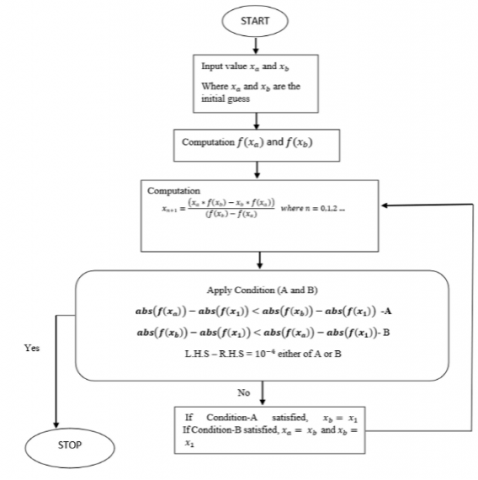Figure 3. Flow chart for modified secant method

4. Application: Problem Formulation

Consider, f(x)=cos(x)-x*exp(x), use the Modified Secant method to solve the problem.

Step 1: Consider two initial guesses, let's say, x-1 and x0.

Let x-1=0.5, 1st initial guess

x0 = 1, 2nd initial guess

f(x-1) =0.0532

f(x0) = -2.1779

Step 2: Put x-1, x0, f(x-1), f(x0) in general the formula is given below.

$x_{i+1}=\frac{\left(x_{i-1} \,\,* f\left(x_i\right)-x_i * f\left(x_{i-1}\,\,\right)\right)}{\left(f\left(x_i\right)-f\left(x_{i-1}\,\,\right)\right.}$

For 1st iteration, i=0

\begin{aligned} x_1 & =\frac{\left(x_{-1} * f\left(x_0\right)-x_0 * f\left(x_{-1}\right)\right)}{\left(f\left(x_0\right)-f\left(x_{0-1}\right)\right.} \\ x_1 & =0.5119 \text { and } f\left(x_1\right)=0.01773\end{aligned}

Step 3: - Use Conditions (A and B) for the 2nd iteration.

If $a b s\left(f\left(x_a\right)\right)-a b s\left(f\left(x_1\right)\right)<a b s\left(f\left(x_b\right)\right)-a b s\left(f\left(x_1\right)\right)$ use xa with x1 for 2nd iteration (A).

Initial guess used $x_a=x_{-1}, \quad x_b=x_0$,  put $f\left(x_{-1}\right)=0.0532$ and $f\left(x_0\right)=-2.1779$ in Condition-A as follows:

$0.03547<2.16017(A)($ Satisfied)

If $a b s\left(f\left(x_b\right)\right)-a b s\left(f\left(x_1\right)\right)<a b s\left(f\left(x_a\right)\right)-a b s\left(f\left(x_1\right)\right)$ use xb with x1 for 2nd iteration (B).

Put $f\left(x_{-1}\right)=0.0532$ and f(x0)= -2.1779 in condition-B as follow:

$2.16017<0.03547(B)($ Not Satisfied $)$

Apply condition-A and the formula given as follows:

$x_2=\frac{\left(x_{-1} \,\,* f\left(x_1\right)-x_1 * f\left(x_{-1}\,\,\right)\right)}{\left(f\left(x_1-f\left(x_{-1}\right)\,\,\right)\right.}$

x2=0.5178 and f(x0) = -0.000129

Step 4: - Stop executing once the left-hand and right-hand side differences in any of the conditions are less than the error. Whether the condition is satisfied or not. Use conditions (A and B) as a stopping criterion.

If  $a b s\left(f\left(x_a\right)\right)-a b s\left(f\left(x_1\right)\right)<a b s\left(f\left(x_b\right)\right)-a b s\left(f\left(x_1\right)\right)$ use xa with x1 for new iteration (A).

Here $x_a=x_0$ and $x_b=x_1$ and $x_1=x_2$

2.1779-0.000129<0.01773-0.000129

2.1777<0.01760 (not satisfied but difference is negligible)

x2=0.5178 is the equation's root.

Consider, f(x)=cos(x)-x* exp(x), same problem solved by standard Secant method.

Let x-1=0.5, 1st initial guess

x0 = 1, 2nd initial guess

f(x-1) =0.0532

f(x0) = -2.1779

i = 0 for the 1st iteration, and the formula becomes as follows:

$x_1=\frac{\left(x_{-1} \,\,* f\left(x_0\right)-x_0 * f\left(x_{-1}\,\,\right)\right)}{\left(f\left(x_0\right)-f\left(x_{0-1}\,\,\right)\right.}$

x1=0.5119 and f(x1)=0.01773

i = 1 for the 2nd iteration, and the formula becomes as follows:

$x_2=\frac{\left(x_0 * f\left(x_1\right)-x_1 * f\left(x_0\right)\right)}{\left(f\left(x_1\right)-f\left(x_0\right)\right.}$

\begin{aligned} & x_0=1, f\left(x_0\right)=-2.1779, x_1=0.5119, f\left(x_1\right) 0.1773 \\ & x_2=0.5158 \text { and } f\left(x_2\right)=0.0059\end{aligned}

i = 2 for the 3rd iteration, and the formula becomes as follows:

$x_3=\frac{\left(x_1 * f\left(x_2\right)-x_2 * f\left(x_1\right)\right)}{\left(f\left(x_2\right)-f\left(x_1\right)\right.}$

\begin{aligned} & x_3=0.5178 \text { and } f\left(x_3\right)=-0.000129 \\ & x_3=0.5178 \text { is the equation's root }\end{aligned}

The number of iterations can be lowered by using the Modified Secant technique.

5. Comparision and Validation

5.1 Comparison and validation 1

Consider f(x)= x3-2*x-5

1st initial guess = 2, 2nd initial guess = 3

Final root = 2.0946

Modified Secant Method = 4 Iteration

Secant Method = 5 Iteration

Regula Falsi Method = 10 Iteration

Bisection Method = 15 Iteration

Newton Raphson Method = 2 Iteration

Values obtained using different root finding methods are tabulated in Table 1 and 2.

Table 1. Comparison of various methods for a lower number of iterations

 Modified Secant Method Secant Method Regula Falsi Method Bisection Method Newton Raphson Method X1=2.0588 X1=2.0588 X1=2.05882 X1=2.5000 X1=2.1000 X2=2.0966 X2=2.0813 X2=2.08126 X2=2.2500 X2=2.0946 X3=2.0945 X3=2.0948 X3=2.08964 X3=2.1250 X4=2.0946 X4=2.0945 X4=2.09274 X4=2.0625 X5=2.0946 X5=2.09388 X5=2.0938 X6=2.09431 X6=2.1094 X7=2.09466 X7=2.1016 X8=2.09452 X8=2.0977 X9=2.09454 X9=2.0957 X10=2.09455 X10=2.0947 X11=2.0942 X12=2.0945 X13=2.0946 X14=2.0945 X15=2.0946

## a.png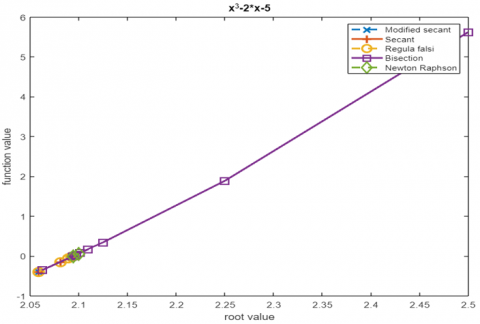(a) Function vs Root value

## b.png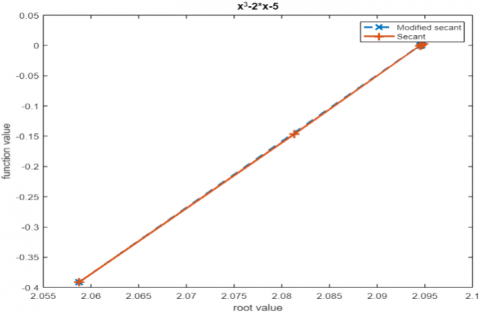(b) Function vs Root value

## c.png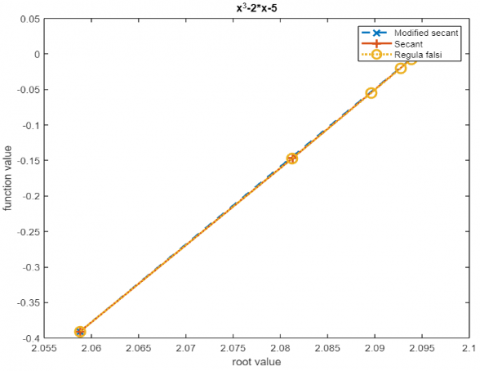(c) Function vs Root value

## d.png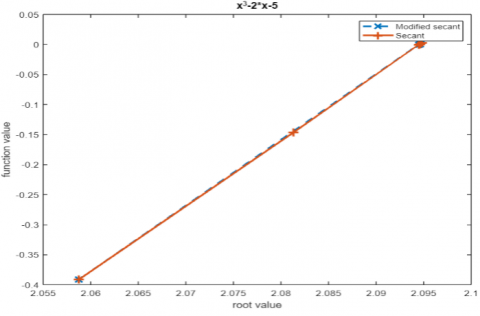(d) Function vs Root value

Figure 4. (a) Function vs Root value; (b) Function vs Root value; (c) Function vs Root value; (d) Function vs Root value

The figures above shows the comparison of root finding method.

Figure 4 (a) shows comparisons between the Modified Secant Method and Standard Secant, Regula Falsi, Newton Raphson and Bisection method for a lower number of iterations.

Figure 4 (b) shows comparisons between the Modified Secant method and Standard Secant, Regula Falsi and Newton Raphson method for the lower number of iterations.

Figure 4 (c) shows comparisons between the Modified Secant method and Standard Secant and Regula Falsi method for the lower number of iterations.

Figure 4 (d) shows comparisons between Modified Secant and Standard Secant methods for lower number of iterations.

From the present study, it is concluded that the Modified secant method has only four iterations, which can be easily found in Figures 4 (a), (b), (c) and (d) when compared with standard secant, Regula Falsi, and the Bisection method. In Figure 4 (a), compared to Newton Raphson's method, the Modified Secant method requires fewer iterations, but not as much.

5.2 Comparison and validation 2

Consider f(x)= cos(x)-x*exp(x)

1st initial guess = 0.5, 2nd initial guess = 1

Final root = 0.5178

Modified Secant method = 2 Iteration

Secant method = 3 Iteration

Regula Falsi method = 8 Iteration

Bisection method = 13 Iteration

Newton Raphson method = 2 Iteration

Table 2. Comparison of various methods for a lower number of iterations

 Modified Secant Method Secant Method Regula Falsi Method Bisection Method Newton Raphson Method X1=0.5119 X1=0.5119 X1=0.51193 X1=0.7500 X1=0.5180 X2=0.5178 X2=0.5159 X2=0.51585 X2=0.6250 X2=0.5178 X3=0.5178 X3=0.51713 X3=0.5652 X4=0.51755 X4=0.5312 X5=0.51769 X5=0.5156 X6=0.51774 X6=0.5234 X7=0.51775 X7=0.5195 X8=0.51776 X8=0.5176 X9=0.5186 X10=0.5181 X11=0.5178 X12=0.5177 X13=0.5178

The figures below shows the comparison of root finding method.

Figure 4 (e) shows the function value against the root value for the Modified Secant Method, Standard Secant, Regula Falsi, Newton Raphson and Bisection method for the lower number of iterations.

Figure 4 (f) shows function value against root value for Modified Secant method, Standard Secant, Regula Falsi and Newton Raphson method for a lower number of iterations

Figure 4 (g) shows function value against root value for Modified Secant method with Standard Secant and Regula Falsi, the method for a lower number of iterations.

Figure 4 (h) shows function value against root value for the Modified Secant method and Standard Secant method for a lower number of iterations.

It can be seen from Figures below that the modified secant method has two iterations, whereas the standard secant method has three iterations. The same can be seen in Figures 4 (e), 4 (f) and 4 (g). The present study concludes that modified secant method conditions (A and B) work on finding those roots which give solution faster than the standard Secant method.

## e.png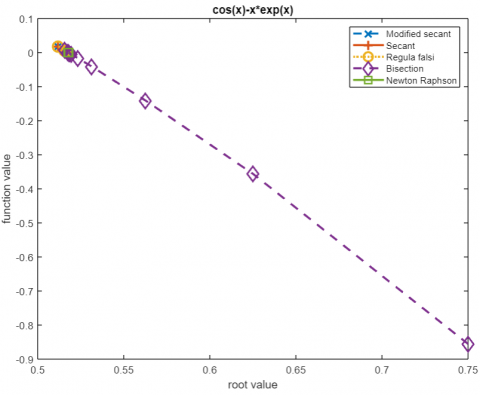(e) Function vs Root value

## f.png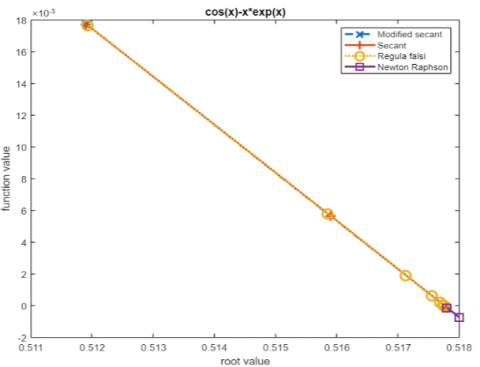(f) Function vs Root value

## g.png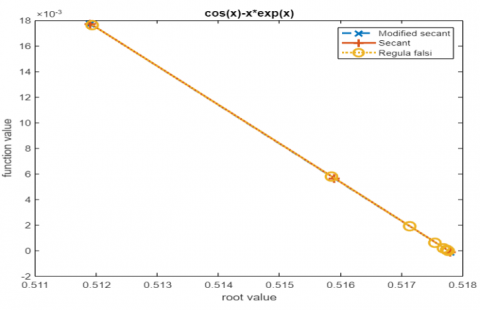(g) Function vs Root value

## h.png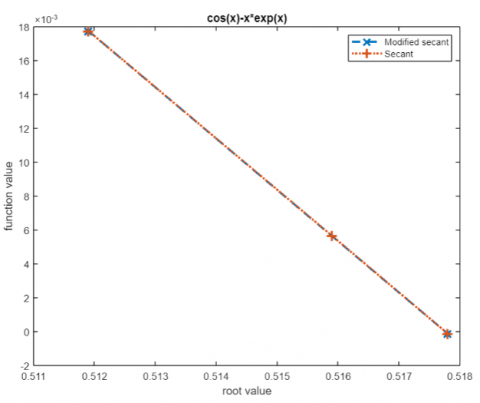(h) Function vs Root value

Figure 4. (e) Function vs Root value; (f) Function vs Root value; (g) Function vs Root value; (h) Function vs Root value

6. Conclusions

The present work shows the Modified Secant approach to locate roots with fewer iterations than the Secant method. This approach can be used to solve any non-linear equation comprising algebraic, transcendental, or other functions. Compared to the Secant, Regula Falsi, and Bisection methods, the approximate root is computed in fewer iterations. For the Modified Secant method, conditions A and B are crucial. The current work identifies these two conditions (A and B). It shows that they satisfied the Secant assertion and clarified using three roots after the first iteration to choose two roots for the second iteration and subsequent iterations. It is also concluded that the Modified Secant method required less computational cost when compared with Bisection, Regula Falsi and the standard Secant method but not with the Newton-Raphson method.

References

 Tiruneh, A.T. (2019). A modified three-point Secant method with improved rate and characteristics of Convergence. https://doi.org/10.48550/arXiv.1902.09058

 Weerakoon, S., Fernando, T.G.I. (2000). A variant of Newton’s method with accelerated third-order convergence. Applied Mathematics Letters, 13(8): 87-93. https://doi.org/10.1016/S0893-9659(00)00100-2

 Grau-Sánchez, M., Díaz-Barrero, J.L. (2011). A technique to composite a modified Newton’s method for solving nonlinear equations. http://arxiv.org/abs/1106.0996

 Kasturiarachi, A.B. (2002). Leap-frogging Newton’s method. International Journal of Mathematical Education in Science and Technology, 33(4): 521-527. https://doi.org/10.1080/00207390210131786

 Magreñán, A., Argyros, I.K. (2016). New improved convergence analysis for the secant method. Mathematics and Computers in Simulation, 119: 161-170. https://doi.org/10.1016/j.matcom.2015.08.002

 Parida, P.K., Gupta, D.K. (2006). An improved Regula-Falsi method for enclosing simple zeros of nonlinear equations. Applied Mathematics and Computation, 177(2): 769-776. https://doi.org/10.1016/j.amc.2005.11.034

 Wang, X., Kou, J., Gu, C. (2010). A new modified secant-like method for solving nonlinear equations. Computers and Mathematics with Applications, 60(6): 1633-1638. https://doi.org/10.1016/j.camwa.2010.06.045

 Wu, Q., Ren, H. (2006). Convergence ball of a modified secant method for finding zero of derivatives. Applied Mathematics and Computation, 174(1): 24-33. https://doi.org/10.1016/j.amc.2005.05.007

 Naghipoor, J., Ahmadian, S.A., Soheili, A.R. (2008). An improved Regula Falsi method for finding simple zeros of nonlinear equations. Applied Mathematical Sciences 2(8): 381-386. https://profdoc.um.ac.ir/articles/a/1016057.pdf

 Livieris, I.E., Pintelas, P. (2013). A new class of spectral conjugate gradient methods based on a modified secant equation for unconstrained optimization. Journal of Computational and Applied Mathematics, 239(1): 396-405. https://doi.org/10.1016/j.cam.2012.09.007

 Amat, S., Busquier, S. (2003). A modified secant method for semismooth equations. Applied Mathematics Letters, 16(6): 877-881. https://doi.org/10.1016/S0893-9659(03)90011-5

 Longley, W.R. (1932). JB Scarborough, Numerical Mathematical Analysis. https://projecteuclid.org/journals/bulletin-of-the-american-mathematical-society/volume-38/issue-5/Review-J-B-Scarborough-Numerical-Mathematical-Analysis/bams/1183495919.pdf, accessed on Jul. 20, 2022.

 Amat, S., Hernández-Verón, M.A., Rubio, M.J. (2014). Improving the applicability of the secant method to solve nonlinear systems of equations. Applied Mathematics and Computation, 247: 741-752. https://doi.org/10.1016/j.amc.2014.09.066

 Ezquerro, J.A., Grau, A., Grau-Sánchez, M., Hernández, M.A., Noguera, M. (2012). Analysing the efficiency of some modifications of the secant method. Computers and Mathematics with Applications, 64(6): 2066-2073. https://doi.org/10.1016/j.camwa.2012.03.105

 Kiusalaas, J. (2015). Numerical Methods in Engineering with MATLAB®. https://doi.org/10.1017/CBO9781316341599

 Grewal, B.S. (2019). Numerical Methods in Engineering and Science.

 Chapra, S.C., Canale, R.P. (2010). Numerical methods for engineers. McGraw-Hill, USA.